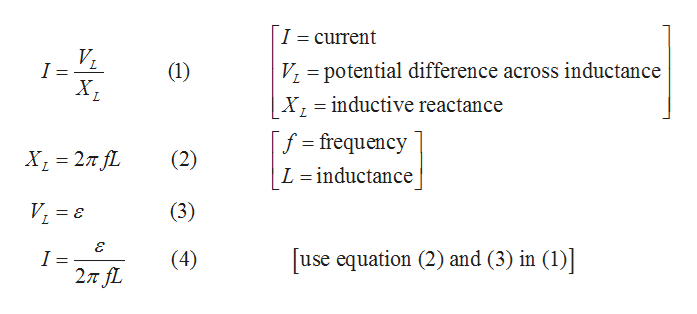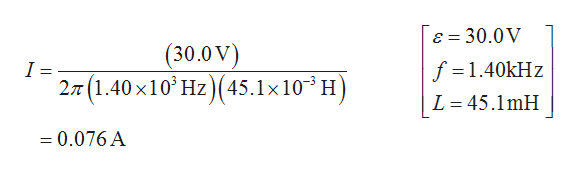Question
8 views

A 45.1 mH inductor is connected as in the figure to an ac generator with εm = 30.0 V. What is the amplitude of the resulting alternating current if the frequency of the emf is (a)1.40 kHz and (b)11.2 kHz?

check_circle

Step 1

(a) Write the expression for the amplitude of the resulting alternating current .help_outlineImage TranscriptioncloseI = current VL I = Vpotential difference across inductance X inductive reactance (1) Х, f frequency х, %3D 2л JL (2) |L inductance V1 (3) use equation (2) and (3) in (1) I = 27 fL (4) fullscreen
Step 2

Substitute the given values  in equation (4)  when the frequency of the emf is 1.40kHzhelp_outlineImage TranscriptioncloseE 30.0V (30.0V) 2T(1.40 x10 Hz) (45.1x103 H f =1.40kHz L 45.1mH =0.076 A fullscreen
Step 3

(b) substitute the given values in equation (4)w...

### Want to see the full answer?

See Solution

#### Want to see this answer and more?

Solutions are written by subject experts who are available 24/7. Questions are typically answered within 1 hour.*

See Solution
*Response times may vary by subject and question.
Tagged in

### Physics